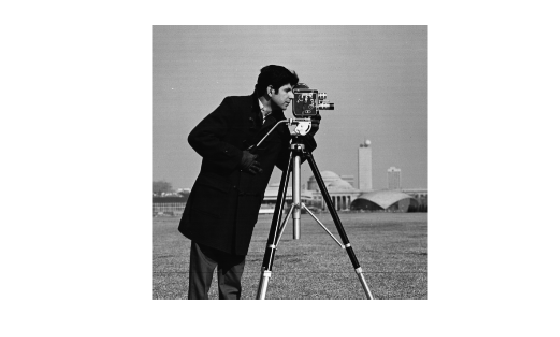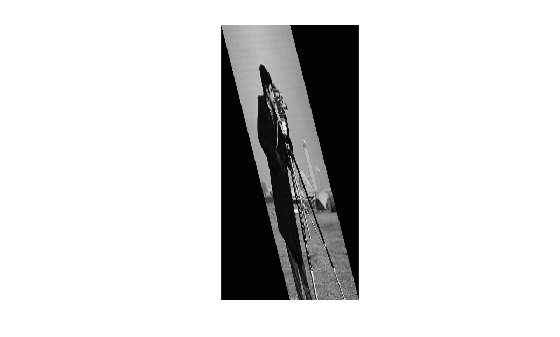# findbounds

Find output bounds for spatial transformation

## Syntax

``outbounds = findbounds(tform,inbounds)``

## Description

example

````outbounds = findbounds(tform,inbounds)` estimates the output bounds corresponding to a given spatial transformation and a set of input bounds. `tform` is a spatial transformation structure. `inbounds` is a 2-by-`num_dims` matrix that specifies the lower and upper bounds of the output image. `outbounds` is an estimate of the smallest rectangular region completely containing the transformed rectangle represented by the input bounds, and has the same form as `inbounds`. Since `outbounds` is only an estimate, it might not completely contain the transformed input rectangle.```

## Examples

collapse all

Read an image into the workspace, and display the image.

```I = imread('cameraman.tif'); figure imshow(I)```Create a spatial transformation structure that stretches an image.

`T = maketform('affine',[.5 0 0; .5 2 0; 0 0 1]);`

Calculate the boundaries of the output image, given the size of the input image and the spatial transformation. The dimensions of the input image are 256-by-256. The boundaries of the output image reflect the transformation: 256-by-512.

`outb = findbounds(T,[0 0;256 256])`
```outb = 2×2 0 0 256 512 ```

Apply the transformation, and display the image.

```transformedI = imtransform(I,T); figure imshow(transformedI)```## Input Arguments

collapse all

Spatial transformation, specified as a structure (`tform`).

Data Types: `struct`

Bounds for each dimension of the input image, specified as a 2-by-`num_dims` matrix. The first row of `inbounds` specifies the lower bounds for each dimension, and the second row specifies the upper bounds. `num_dims` has to be consistent with the `ndims_in` field of `tform`.

Example: `outb = findbounds(T,[0 0;256 256])` where input image is 256-by-256.

Data Types: `double`

## Output Arguments

collapse all

Bounds for each dimension of the output image (output space bounding box), returned as a 2-by-`num_dims` matrix of class `double`.

## Algorithms

1. `findbounds` first creates a grid of input-space points. These points are at the center, corners, and middle of each edge in the image.

```I = imread('rice.png'); h = imshow(I); set(h,'AlphaData',0.3); axis on, grid on in_points = [ ... 0.5000 0.5000 0.5000 256.5000 256.5000 0.5000 256.5000 256.5000 0.5000 128.5000 128.5000 0.5000 128.5000 128.5000 128.5000 256.5000 256.5000 128.5000]; hold on plot(in_points(:,1),in_points(:,2),'.','MarkerSize',18) hold off```

Grid of Input-Space Points2. Next, `findbounds` transforms the grid of input-space points to output space. If `tform` contains a forward transformation (a nonempty `forward_fcn` field), then `findbounds` transforms the input-space points using `tformfwd`. For example:

```tform = maketform('affine', ... [1.1067 -0.2341 0; 0.5872 1.1769 0; 1000 -300 1]); out_points = tformfwd(tform, in_points)```
``` out_points = 1.0e+03 * 1.0008 -0.2995 1.1512 0.0018 1.2842 -0.3595 1.4345 -0.0582 1.0760 -0.1489 1.1425 -0.3295 1.2177 -0.1789 1.2928 -0.0282```

If `tform` does not contain a forward transformation, then `findbounds` estimates the output bounds using the Nelder-Mead optimization function `fminsearch`.

3. Finally, `findbounds` computes the bounding box of the transformed grid of points.

## Version History

Introduced before R2006a